# An experiment consists of rolling a weighted die. The probability of rolling each number is: Pr=0.05Pr=0.05, Pr=0.25Pr=0.25, Pr=0.1Pr=0.1, Pr=0.15Pr=0.15, Pr=0.2Pr=0.2, and Pr=0.25Pr=0.25. On the first roll, you record if the number is Small (1,2) or Large (3,4,5,6). If the first number is Small, then on the second roll you record if the number is a 1 or not. If the first number is Large, on the second roll you record whether the number is Small or not. So, some typical outcomes would be S1 (small number rolled, then 1) or LL (large number rolled, then another large number). Draw a tree diagram and use it to answer the following questions.(1) What is Pr[S1]?(2) What is Pr[LL]? (3) What is Pr[(not1)onsecondroll|Sonfirstroll]?(4) What is Pr[S on second roll|L on first roll]?

Question
25 views

An experiment consists of rolling a weighted die. The probability of rolling each number is: Pr=0.05Pr=0.05, Pr=0.25Pr=0.25, Pr=0.1Pr=0.1, Pr=0.15Pr=0.15, Pr=0.2Pr=0.2, and Pr=0.25Pr=0.25. On the first roll, you record if the number is Small (1,2) or Large (3,4,5,6). If the first number is Small, then on the second roll you record if the number is a 1 or not. If the first number is Large, on the second roll you record whether the number is Small or not. So, some typical outcomes would be S1 (small number rolled, then 1) or LL (large number rolled, then another large number). Draw a tree diagram and use it to answer the following questions.

(1) What is Pr[S1]?

(2) What is Pr[LL]?

(3) What is Pr[(not1)onsecondroll|Sonfirstroll]?

(4) What is Pr[S on second roll|L on first roll]?

check_circle

star
star
star
star
star
1 Rating
Step 1

The tree diagram for the given information is drawn as follows: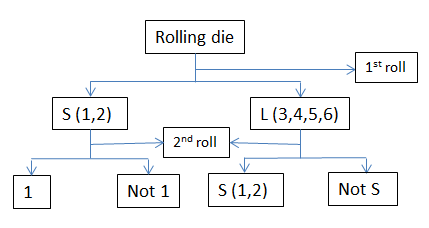It is given that,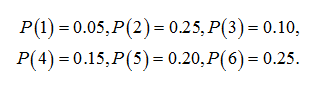Step 2

Consider,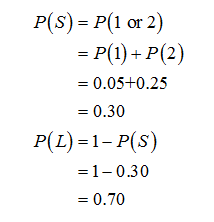Step 3

1).

The probability of the event P(S1) is calculated as follows: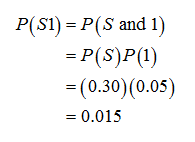2).

The probability of the event P(LL) is calculated as follows: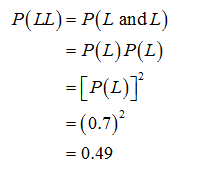...

### Want to see the full answer?

See Solution

#### Want to see this answer and more?

Solutions are written by subject experts who are available 24/7. Questions are typically answered within 1 hour.*

See Solution
*Response times may vary by subject and question.
Tagged in

### Probability